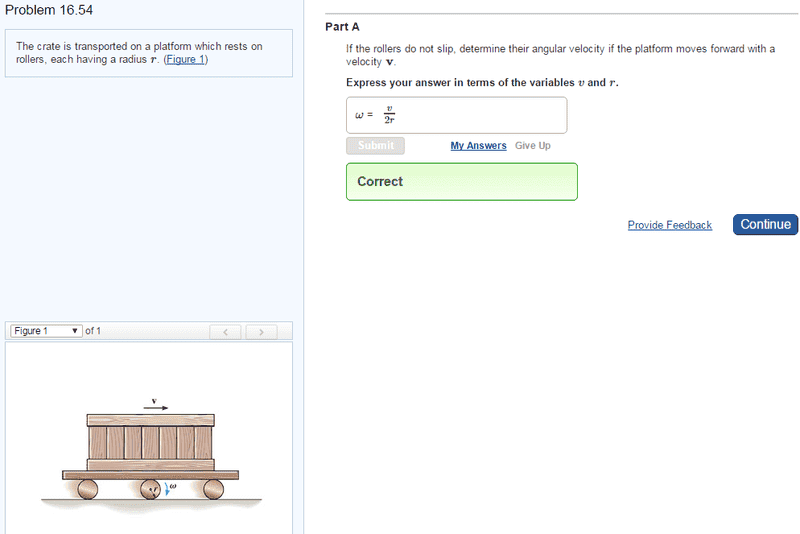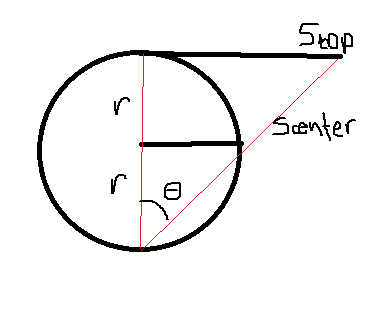# Angular Velocity Calculation

## Homework Statementv = w x r

## The Attempt at a Solution

I got the answer correct after a few tries, but I am little confused on why it has to solved this specific way. I know that v = w x r, so why can I not just say w = v/r? Is it because w is measured from the center of the roller so v = w x r would be vcenter, not vtop?
That is how I ended up solving it, but I wanted to make sure I didn't get there "accidentally" or misinterpreted the process.Using that diagram and concept of similar triangles:
tan θtop = tan θcenter
Stop/2r = Scenter/r
Stop = 2*Scenter, Scenter = rθ
Stop = 2rθ
d(Stop)/dt = vtop = d(2rθ)/dt = 2rθ' = 2rωtop
thus, ω = v/2r

Any reasoning as to why I have to do this would be much appreciated!!